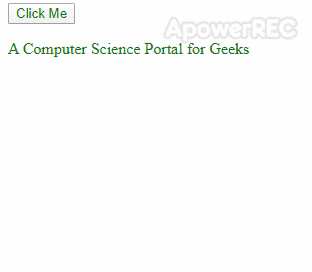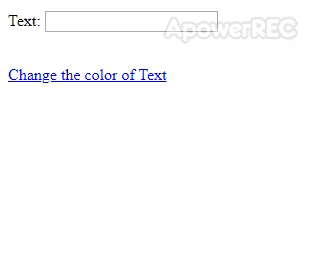GeeksforGeeks App
Open AppBrowser
Continue

# How to use JavaScript variables in jQuery selectors ?

In this article, we will discuss how to use JavaScript variables in jQuery selectors. In the following examples, it can be seen that we have used the values stored in JavaScript Variables used inside the jQuery Selectors.

Example 1: The concatenation technique can be applied in order to use the values stored in JavaScript variables. In the following example, whenever the button is clicked, the content present inside the <span> element is appended to the <p> element. Then we will use the ready() method that helps to load the whole page and then execute the rest code.

## html

 `` `<``html``>` `<``head``>``    ``<``title``>JavaScript variables in jQuery slectors` `    ``<``script` `src="https://code.jquery.com/jquery-3.4.1.js"``            ``integrity=``"sha256-WpOohJOqMqqyKL9FccASB9O0KwACQJpFTUBLTYOVvVU="``            ``crossorigin="anonymous">``    ````` `<``body``>``    ``<``div``>``        ``<``button` `id="button"``                ``style="color: green;">``            ``Click Me``        ``` `        ``<``p` `id="firstpara">``            ``<``span` `id="span"``                  ``style="color: green;">``                ``A Computer Science Portal for Geeks<``br``>``            ````        ````    ````    ``<``script``>``        ``\$(document).ready(function() {``            ``\$("#button").click(function() {``                ``var paraId = "firstpara";``                ``var spanId = "span";``                ``\$("#" + paraId).append(\$("#" + spanId).html());``            ``});``        ``})``    ````` ``

Output:Example 2: The following example changes the color of the text when the link is pressed. In this example, javascript:void(0); is used inside <a> element. Then we will use the ready() method helps to load the whole page and then execute the rest code.

## html

 ```<``html``>` `<``head``>``    ``<``title``>JavaScript variables in jQuery slectors` `    ``<``script` `src="https://code.jquery.com/jquery-3.4.1.js"``            ``integrity=``"sha256-WpOohJOqMqqyKL9FccASB9O0KwACQJpFTUBLTYOVvVU="``            ``crossorigin="anonymous">``    ````` `<``body``>``    ``<``form``>``        ``<``p``>``          ``Text:``          ``<``input` `type="text">``        ````        ``<``br``>` `        ``<``a` `href="javascript:void(0);">``          ``Change the color of Text``        ````    ````    ``<``script``>``        ``\$(document).ready(function() {` `            ``\$("a").click(function() {``                ``var type = \$("input").attr("type");``                ``var attribute = "color";``                ``var color = "green";` `                ``\$("input[type=" + type + "]")``                ``.css(attribute, color);``            ``});``        ``})``    ````` ``

Output:jQuery is an open-source JavaScript library that simplifies the interactions between an HTML/CSS document, It is widely famous for its philosophy of “Write less, do more”. You can learn jQuery from the ground up by following this jQuery Tutorial and jQuery Examples.

My Personal Notes arrow_drop_up
Related Tutorials